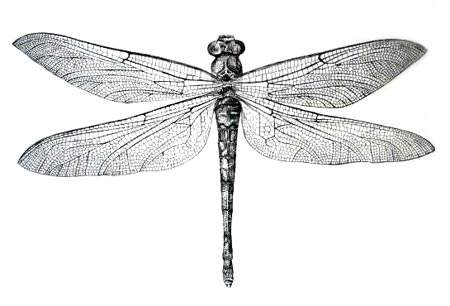## 网格噪声（Cellular Noise）

1996 年，在原始的 Perlin Noise 发布六年后，Perlin 的 Simplex Noise 发布五年前，Steven Worley 写了一篇名为“A Cellular Texture Basis Function”的论文。在这篇论文里，他描述了一种现在被广泛使用的程序化纹理技术。

``````    float min_dist = 100.; // A variable to store the closest distance to a point

min_dist = min(min_dist, distance(st, point_a));
min_dist = min(min_dist, distance(st, point_b));
min_dist = min(min_dist, distance(st, point_c));
min_dist = min(min_dist, distance(st, point_d));``````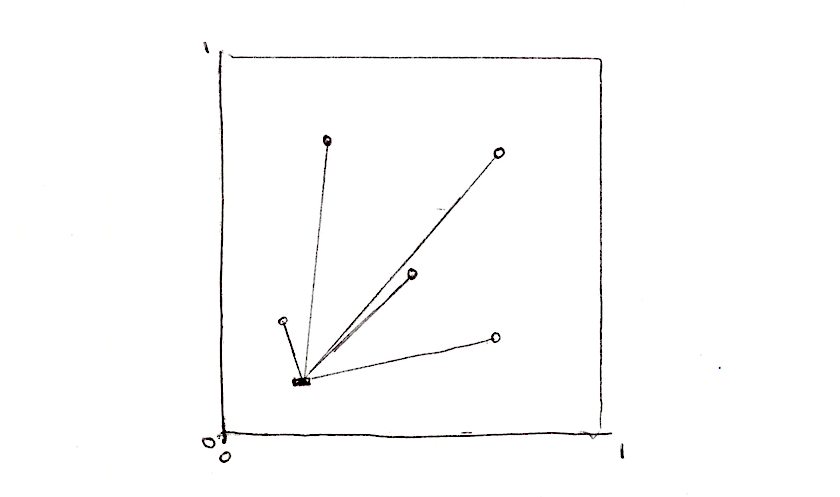``````    float m_dist = 100.;  // minimum distance
for (int i = 0; i < TOTAL_POINTS; i++) {
float dist = distance(st, points[i]);
m_dist = min(m_dist, dist);
}``````

• 你可以让其余的几个特征点也动起来吗？
• 在读完关于形状的章节后，想象一些关于距离场的有意思的用法。
• 如果你要往距离场里添加更多的特征点怎么办？如果我们想动态地添加减少特征点数怎么办？

### 平铺和迭代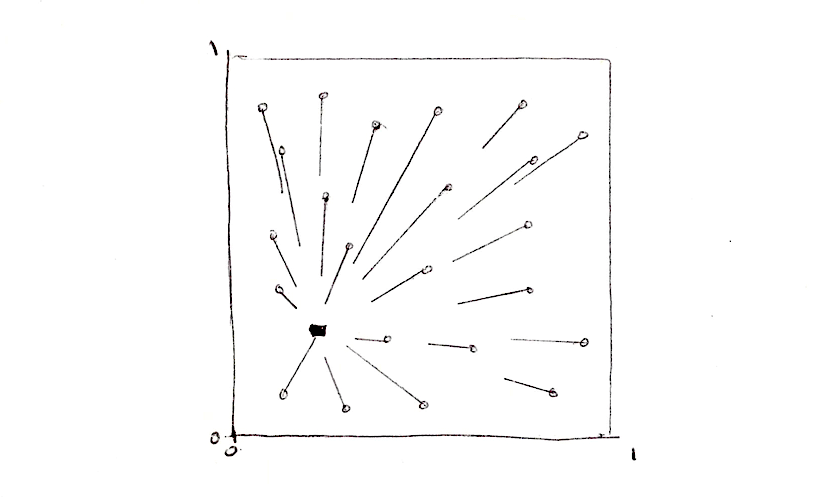``````    // Scale
st *= 3.;

// Tile the space
vec2 i_st = floor(st);
vec2 f_st = fract(st);``````

``    vec2 point = random2(i_st);``

``````    vec2 diff = point - f_st;
float dist = length(diff);``````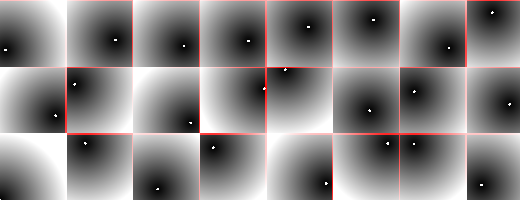``````for (int y= -1; y <= 1; y++) {
for (int x= -1; x <= 1; x++) {
// Neighbor place in the grid
vec2 neighbor = vec2(float(x),float(y));
...
}
}``````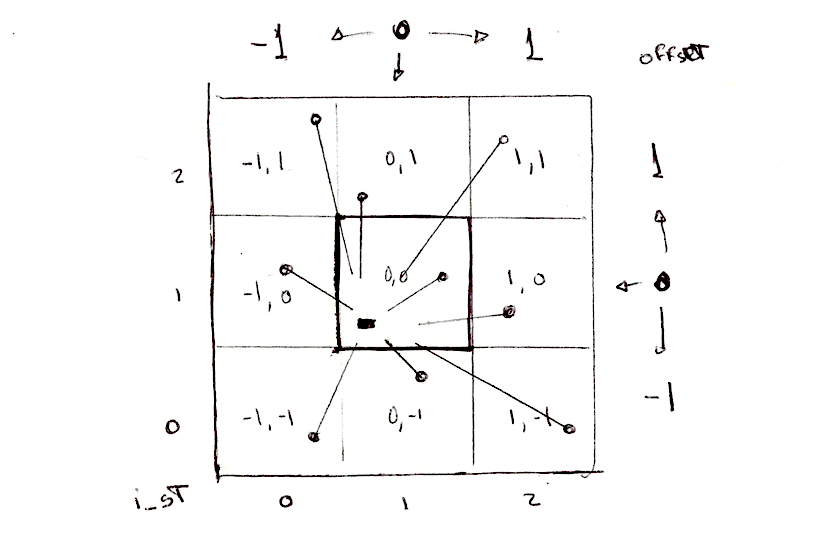``````        ...
// Random position from current + neighbor place in the grid
vec2 point = random2(i_st + neighbor);
...``````

``````        ...
vec2 diff = neighbor + point - f_st;

// Distance to the point
float dist = length(diff);

// Keep the closer distance
m_dist = min(m_dist, dist);
...``````

“可能值得注意的是，上面的代码中有一个很漂亮的技巧。多数实现都存在精度问题，因为他们是在“域”空间（如“世界”或“对象”空间）内产生随机点，这可能是里原点任意远的。要解决这个问题，可以使用更高精度的数据类型，或更聪明些。我的实现不是在“域”空间（如“世界”或“对象”空间）内产生随机点，而是在“网格”空间内：一旦提取了着色点的整数和小数部分，我们当前的网格就确定了，我们所关心的就是这个网格周围发生了什么，意味着我们可以将所有坐标的整数部分放在一起，从而节省了许多精度位。事实上，一个常规的 voronoi 实现中，从着色点减去随机特征点时，点坐标的整数部分简单地消除掉了。上面的实现中，我们甚至不会让这种消除发生，因为我们正在把所有的计算移到“网格”空间。这个技巧可以让你处理这种情况： 你想要把 voronoi 用在整个星球上——可以简单地将输入替换为双精度，执行 floor() 和 fract() 计算，其余的计算仍使用浮点数，省去了将整个实现改成双精度的成本。当然，同样的技巧也适用于 Perlin Noise 模式（但是我还没有在任何地方看到过它的实现或记录）。”

• 缩放空间。
• 你有其它办法让那些特征点动起来吗？
• 如果我们想要加入一个鼠标位置作为其中一个特征点怎么办？
• 有没有其它办法构造这个距离场，除了 `m_dist = min(m_dist, dist);` 之外？
• 用这个距离场你可以创造出什么有意思的图案？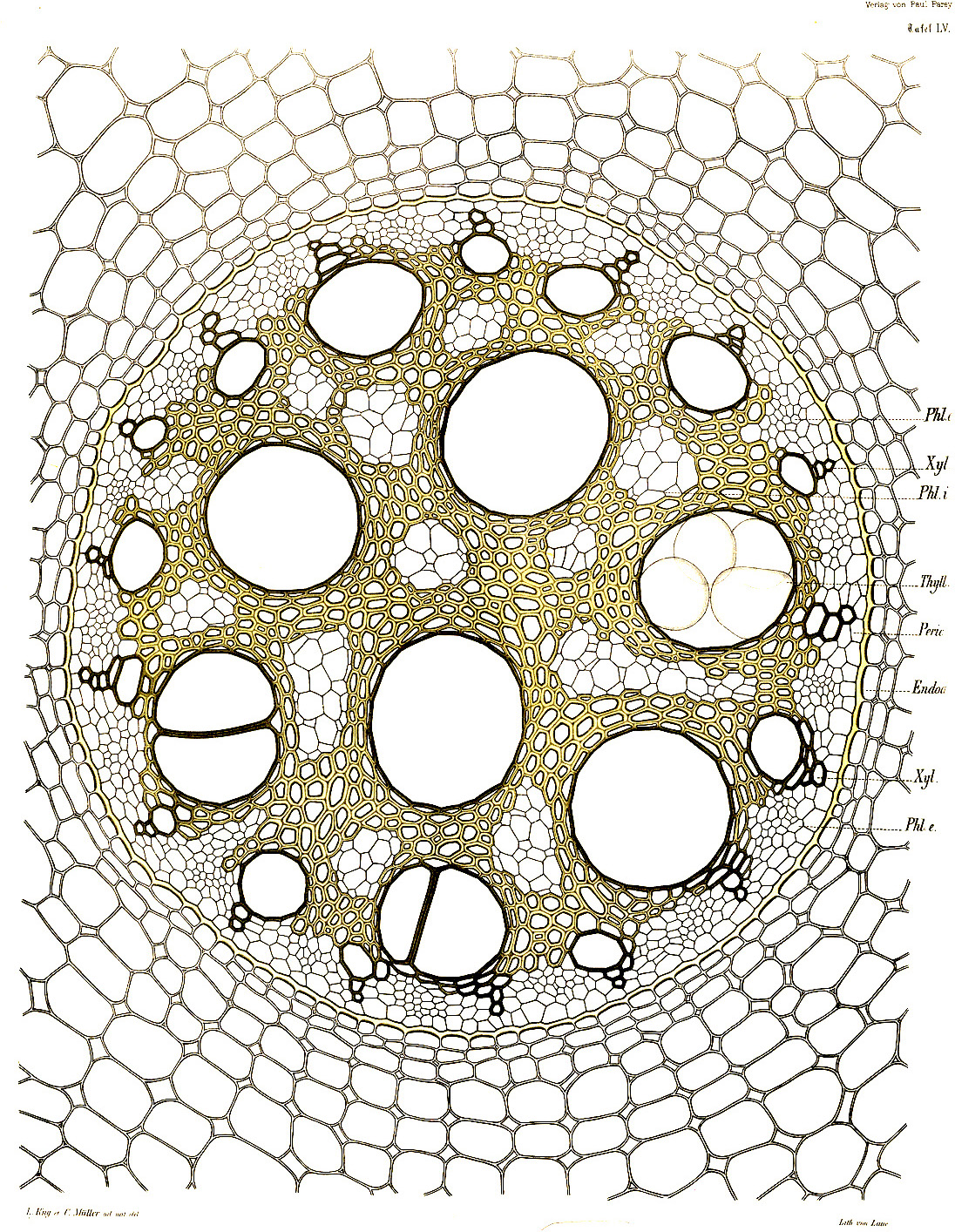### Voronoi 算法

``````    ...
if( dist < m_dist ) {
m_dist = dist;
m_point = point;
}
...``````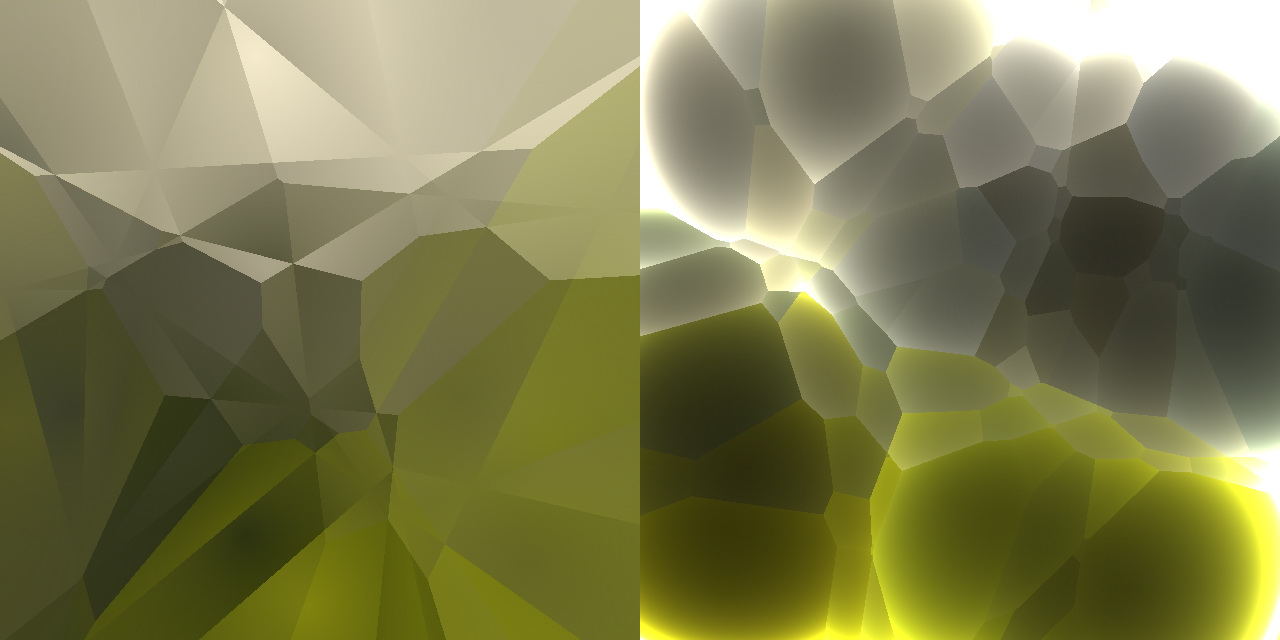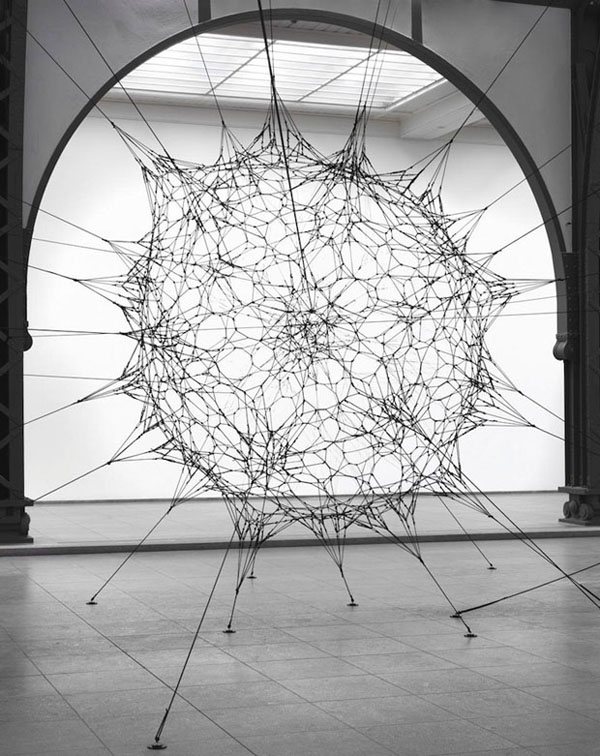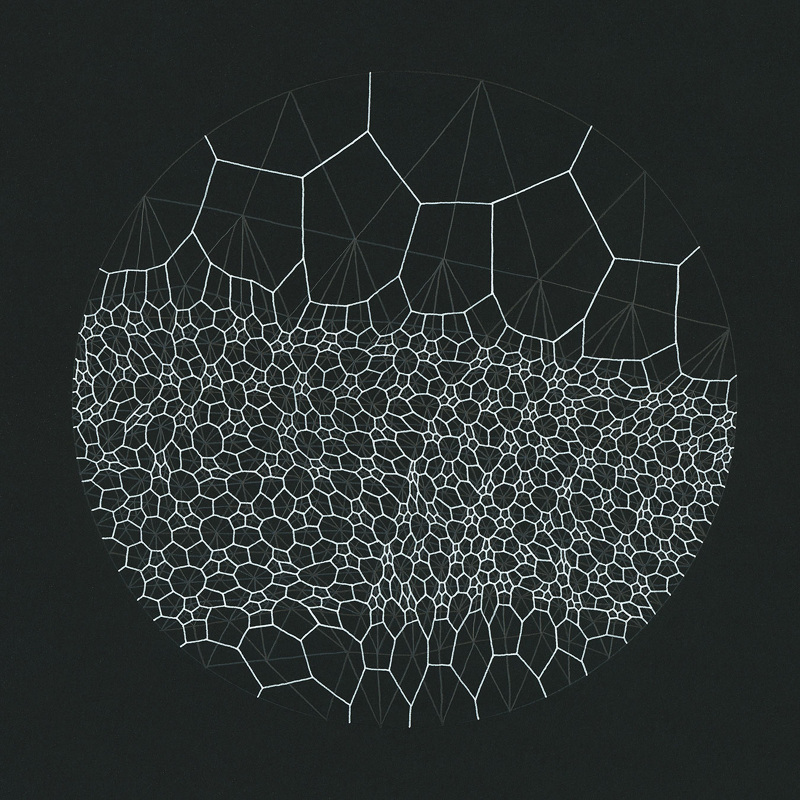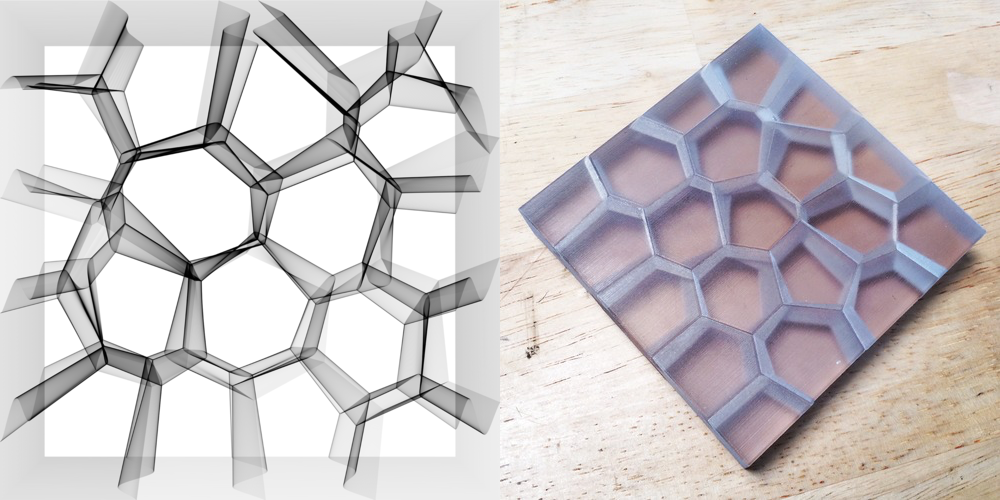### 优化 Voronoi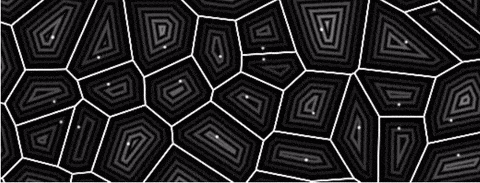Inigo 在 Voronoi 上的实验并没有就此停止。2014 年，他写了一篇非常漂亮的文章，提出一种他称作为 voro-noise 的噪声，可以让常规噪声和 voronoi 逐渐地融合。用他的话说：

“尽管有这样的相似之处，但事实上，两种模式中网格的使用方式都是不同的。噪声会内插或平均随机值（如值噪声），而 Voronoi 是计算到最近特征点的距离。平滑双线性插值和最小值评估是两个非常不同的操作，或者……它们是否能用更广义的方法组合？如果是这样，那么噪声和 Voronoi 模式都可以被看作是一种更一般的以网格为基础的模式生成器？”